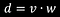# Math in Unity : Dot Product (Part I)

Let’s continue our “mathematical” journey talking about the DoT Product.
First, WHAT IS THE DOT PRODUCT?

# The Dot Product is the sum of the multiplication of the components of a Vector.

In mathematics the written formula is this:The small square between the v and the w is the mathematical symbol of the Dot

Let’s take an example to better understand:
if we have two Vectors V (3, 9) and W (2, 7), applying the Dot formula the result is this:

# An important thing to know is that even if we are doing calculations between Vectors, the result, that is the Dot Product, IS NOT A VECTOR BUT A REAL NUMBER.

Obviously the question is: what does that number represent?
We explain it immediately:

# This is the rule :If the Dot Product is > 0, the angle is < 90°If the Dot Product = 0, the angle is perfectly at 90°if the Dot Product < 0, the angle is > 90°

Now, even now that we have established this simple rule, the question remains: how can we use the Dot Product “in practice”?

A very common use is to establish the FORWARD DIRECTION of a GameObject respect to another.

For example, if an enemy is in FRONT, the Dot Product will be GREATER than 0, while if the enemy is BEHIND, the Dot Product will be LESS than 0.

This concept applies very well in situations where we want an object to ROTATE its front view towards another object.

For example, if we have the Player in one position and the Enemy in another, to make the Player rotate TOWARDS THE ENEMY we need to calculate the angle between OUR FRONT VIEW and the Enemy’s position, ok?

Right now let’s talk about Trigonometry.
The relationship formula between the Dot Product and the angle is this:

The equation starting from the angle instead is this:

As always do not panic, the first impression is always the worst in these cases but if we break this formula into small pieces it is easy to understand and use it for our purposes.

# The angle = inverse cosine of the Dot Product divided by the length of the two Vectors.

Seeing these things in practice is definitely the best thing to do, but a little theory, in my opinion, can really help to better understand how certain mechanisms work and how we can use them.

Note : We always remember that we are implementing these functions from scratch to understand how these calculations work, but they are ALREADY PART OF THE UNITY, precisely in the Vector3 Class.

I leave you the reference of the Dot Product below
https://docs.unity3d.com/ScriptReference/Vector3.Dot.html

In the next article we will see exactly how to put these principles into practice.

Always in love with programming, even if late (I'm already 39 years old) I decided to follow my dream! We will see how far my passion will take me!

## More from Matteo Lo Piccolo

Always in love with programming, even if late (I'm already 39 years old) I decided to follow my dream! We will see how far my passion will take me!